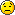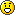# A simple program help???

Status
Not open for further replies.

#### Zangetsu

##### I am the master of my Fate.
Hi Guys,If have a problem in solving this & i googled but cudn't find ne solution...

if integer a = 12345. write a program (without using any in-built function) in C++/C# or
VB.NET. to find the length of integer a (obviously in this case it is 5).remember if its array then its simple.but its an integer datatype.

pls solve my problem?#### Vishal Patil

##### Linux all the way
int a=12345;
int count = 0;
while(a !=0 )
{
a = a/10; //removes the last digit of the number; or better a /= 10
count++;
}

after the loop is executed a will be zero count will contain the number of digits in the number. if you want back a just use int b = a; //before the loop a =b; //and after the loop

Last edited:

#### lucifer_is_back

##### Journeyman
Code:
``````int a=12345;
if(a<0)a=-a;
int count = 0;
while(a !=0 )
{
a = a/10; //removes the last digit of the number; or better a /= 10
count++;
}``````
check for negative

#### Vishal Patil

##### Linux all the way
does work. the condition is a ! = 0; not a < 0

#### Zangetsu

##### I am the master of my Fate.
Ohhh! so simple, Thanks Vishalok...if i want 2 reverse the digit then like o/p= 54321 in the same program#### Vishal Patil

##### Linux all the way
int a=12345;
if(a<0)a=-a;
int count = 0;
int remainder, reverse=0; // ----change over here
while(a !=0 )
{

remainder = a%10; // to read the last digit -----change

a = a/10; //removes the last digit of the number; or better a /= 10

reverse = (reverse * 10) + remainder; //this statement should come before count++ else the value will be 543210

count++;
}

#### Zangetsu

##### I am the master of my Fate.
int a=12345;
if(a<0)a=-a;
int count = 0;
int remainder, reverse=0; // ----change over here
while(a !=0 )
{

remainder = a%10; // to read the last digit -----change

a = a/10; //removes the last digit of the number; or better a /= 10

reverse = (reverse * 10) + remainder; //this statement should come before count++ else the value will be 543210

count++;
}

Oh..thank u very much...but i think for the above program count is not needed...Status
Not open for further replies.